Magnetic Effect Of Current MCQ Level - 1

# Magnetic Effect Of Current MCQ Level - 1

Test Description

## 10 Questions MCQ Test Topic wise Tests for IIT JAM Physics | Magnetic Effect Of Current MCQ Level - 1

Magnetic Effect Of Current MCQ Level - 1 for Physics 2022 is part of Topic wise Tests for IIT JAM Physics preparation. The Magnetic Effect Of Current MCQ Level - 1 questions and answers have been prepared according to the Physics exam syllabus.The Magnetic Effect Of Current MCQ Level - 1 MCQs are made for Physics 2022 Exam. Find important definitions, questions, notes, meanings, examples, exercises, MCQs and online tests for Magnetic Effect Of Current MCQ Level - 1 below.
Solutions of Magnetic Effect Of Current MCQ Level - 1 questions in English are available as part of our Topic wise Tests for IIT JAM Physics for Physics & Magnetic Effect Of Current MCQ Level - 1 solutions in Hindi for Topic wise Tests for IIT JAM Physics course. Download more important topics, notes, lectures and mock test series for Physics Exam by signing up for free. Attempt Magnetic Effect Of Current MCQ Level - 1 | 10 questions in 30 minutes | Mock test for Physics preparation | Free important questions MCQ to study Topic wise Tests for IIT JAM Physics for Physics Exam | Download free PDF with solutions
 1 Crore+ students have signed up on EduRev. Have you?
Magnetic Effect Of Current MCQ Level - 1 - Question 1

### A current i is flowing in a straight conductor of length L. The magnetic induction at a point distant L/4 from its centre will be :

Detailed Solution for Magnetic Effect Of Current MCQ Level - 1 - Question 1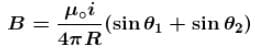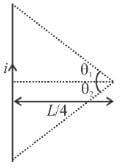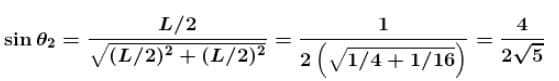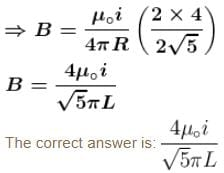Magnetic Effect Of Current MCQ Level - 1 - Question 2

### A long solenoid carrying a current produce’s a magnetic field B along its axis. If the current is doubled acid the number of turns per cm  is halved, the new value of the magnetic field is :

Detailed Solution for Magnetic Effect Of Current MCQ Level - 1 - Question 2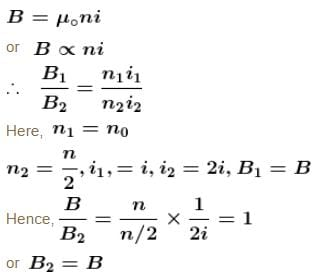The correct answer is: B

Magnetic Effect Of Current MCQ Level - 1 - Question 3

### For paramagnetic materials magnetic susceptibility is related with temperature as :

Detailed Solution for Magnetic Effect Of Current MCQ Level - 1 - Question 3

For paramagnetic materials magnetic susceptibility is inversely proportional to the temperature i.e., proportional to  T–1.
The correct answer is: ∝T-1

Magnetic Effect Of Current MCQ Level - 1 - Question 4

An electron and proton enter a uniform magnetic field perpendicular. Both have same kinetic energy. Which of the following is true?

Detailed Solution for Magnetic Effect Of Current MCQ Level - 1 - Question 4

When a charged particle enters a magnetic field perpendicularly, it moves on a circular path. The required centripetal force is provided by magnetic force.

i.e. magnetic force = Centripetal force.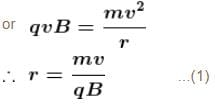Now kinetic energy of the particle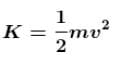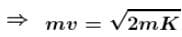Therefore, Eq. (1) become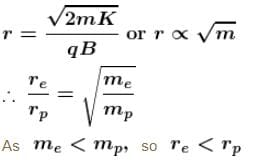Hence trajectory of proton is less curved.

The correct answer is: Trajectory of proton is less curved

Magnetic Effect Of Current MCQ Level - 1 - Question 5

A charge of 10–6C is describing a circular path of radius 1cm making 5 revolution per second.  The magnetic induction field at the centre of the circle is :

Detailed Solution for Magnetic Effect Of Current MCQ Level - 1 - Question 5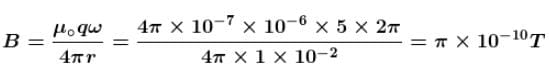The correct answer is: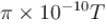Magnetic Effect Of Current MCQ Level - 1 - Question 6

A charged particle enters a uniform magnetic field with velocity vector at an angle of 45° with the magnetic field. The pitch of the helical path followed by the particle is  p. The radius of the helix will be :

Detailed Solution for Magnetic Effect Of Current MCQ Level - 1 - Question 6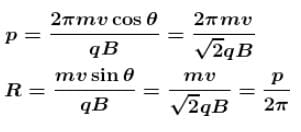The correct answer is: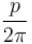Magnetic Effect Of Current MCQ Level - 1 - Question 7

A charged particle (charge  q) is moving in a circle of radius R with uniform speed v. The associated magnetic moment µ is given by :

Detailed Solution for Magnetic Effect Of Current MCQ Level - 1 - Question 7

As revolving charge is equivalent to a current, so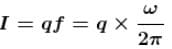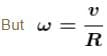where R is radius of circle and v is uniform speed of charged particle.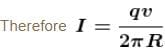Now, magnetic moment associated with charged particle is given by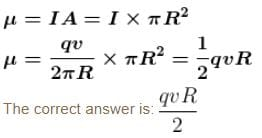Magnetic Effect Of Current MCQ Level - 1 - Question 8

The magnetic induction at centre O  in the following figure will be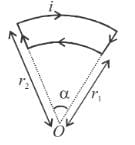Detailed Solution for Magnetic Effect Of Current MCQ Level - 1 - Question 8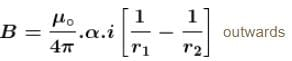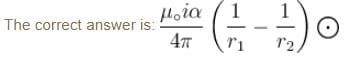Magnetic Effect Of Current MCQ Level - 1 - Question 9

A current carrying wire is bent in the L shape as shown in figure. The length of both arms extend to infinity. Then, the magnetic field at O is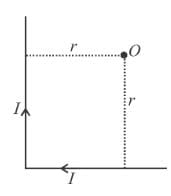Detailed Solution for Magnetic Effect Of Current MCQ Level - 1 - Question 9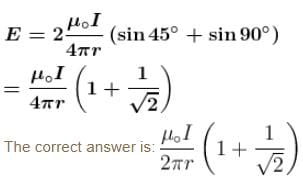Magnetic Effect Of Current MCQ Level - 1 - Question 10

A current loop consists of two identical semicircular parts each of radius R, one lying in the x - y plane and the other in x - z plane. If the current in the loop is i. The resultant magnetic field due to the two semiconductor parts at their common centre is :

Detailed Solution for Magnetic Effect Of Current MCQ Level - 1 - Question 10

The loop mentioned in the question must look like one as shown in the figure.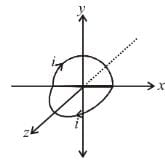Magnetic field at the centre due to semicircular loop lying in x-y plane.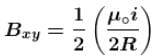in negative z direction.

Similarly field due to loop in x-z plane,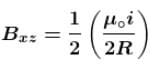in negative y direction.

∴ Magnetic of resultant magnetic field,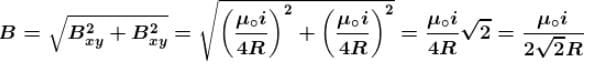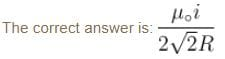## Topic wise Tests for IIT JAM Physics

217 tests
 Use Code STAYHOME200 and get INR 200 additional OFF Use Coupon Code
Information about Magnetic Effect Of Current MCQ Level - 1 Page
In this test you can find the Exam questions for Magnetic Effect Of Current MCQ Level - 1 solved & explained in the simplest way possible. Besides giving Questions and answers for Magnetic Effect Of Current MCQ Level - 1, EduRev gives you an ample number of Online tests for practice

217 tests